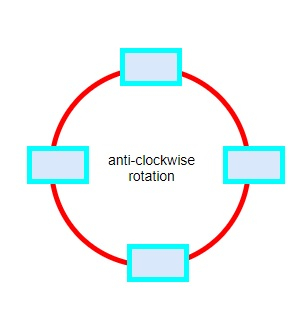# Program to find out the minimum rotations needed to maximize the profit from a Ferris wheel in Python

Suppose there is a Ferris wheel with four cabins and each cabin can contain four passengers. The wheel rotates counter-clockwise, and for each rotation, it costs 'run' amount of money. We now have an array 'cust' that contains n items and each item i signifies the number of people waiting to get into the Ferris wheel before the i-th rotation. To board the wheel, each customer has to pay an amount of money 'board', and that much money is for one anti-clockwise rotation of the wheel. The people waiting in line should not wait if there is any vacant seat available in any cabin. So given the data, we have to find out the minimum amount of rotations required so that the profit can be maximized.So, if the input is like cust = [6,4], board = 6, run = 4, then the output will be 3

At first, 6 people are waiting in line. So at first 4 people get into the first cabin, and the rest wait for the next cabin.

The wheel rotates, and the second cabin arrives. Meanwhile, 4 more people get into the line. So the next 4 people waiting get into the next cabin.

The wheel rotates again, and the remaining three customers get into the next cabin.

So, three rotations are needed in minimum to serve all customers.

The maximum profit achievable from these rotations is (10 * 6) - (3 * 4) = 48.

To solve this, we will follow these steps −

• res := -1

• mst := 0

• tmp := 0

• wt := 0

• for each index idx and value val in cust, do

• wt := wt + val

• chg := minimum of (4, wt)

• wt := wt - chg

• tmp := tmp + chg * board - run

• if mst < tmp, then

• res := idx + 1

• mst := tmp

• x := wt / 4

• y := wt mod 4

• if 4 * board > run, then

• res := res + x

• if y * board > run, then

• res := res + 1

• return res

## Example

Let us see the following implementation to get better understanding

def solve(cust, board, run):
res = -1
mst = 0
tmp = 0
wt = 0
for idx, val in enumerate(cust):
wt += val
chg = min(4, wt)
wt -= chg
tmp += chg * board - run
if mst < tmp:
res, mst = idx+1, tmp
x, y = divmod(wt, 4)
if 4 * board > run:
res += x
if y * board > run:
res += 1
return res

print(solve([6,4], 6, 4))

## Input

[6,4], 6, 4


## Output

3